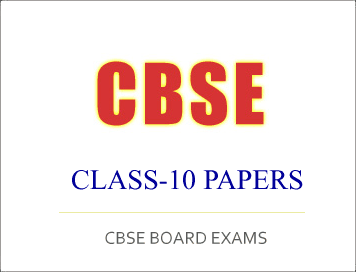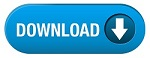NEW!  CBSE Papers PDF: Class-X, Class-XII

# (Download) CBSE Class-10 Sample Paper And Marking Scheme 2020-21 : Mathematics Basic

Disclaimer: This website is not at associated with CBSE, For official website of CBSE visit - www.cbse.nic.in## Mathematics-Basic

Class- X Mathematics-Basic (241)

Sample Question Paper 2020-21

Max. Marks: 80

Duration:3 hours

General Instructions:

1.  This question paper contains two parts A and B.

2.  Both Part A and Part B have internal choices.

Part – A:

1.  It consists of two sections- I and II

2.  Section I has 16 questions. Internal choice is provided in 5 questions.

3.  Section II has four case study-based questions. Each case study has 5 case-based sub-parts. An examinee is to attempt any 4 out of 5 sub-parts.

Part – B:

1.  Question No 21 to 26 are Very short answer Type questions of 2 mark each,

2.  Question No 27 to 33 are Short Answer Type questions of 3 marks each

3.  Question No 34 to 36 are Long Answer Type questions of 5 marks each.

4.  Internal choice is provided in 2 questions of 2 marks, 2 questions of 3 marks and 1 question of 5 marks.

 Questi on No. Part-A Marks Section-I 1. Express 156 as the product of primes. 1 2. Write a quadratic polynomial, sum of whose zeroes is 2 and product is -8. 1 3. Given that HCF (96,404) is 4, find the LCM ( 96,404). OR State the fundamental Theorem of Arithmetic. 1
 4 On comparing the ratios of the coefficients, find out whether the pair of equations x – 2y =0 and 3x + 4y -20 =0 is consistent or inconsistent. 1 5 If a and b are co-prime numbers, then find the HCF (a, b). 1 6 Find the area of a sector of a circle with radius 6cm if angle of the sector is 60°. (Take ?? = 22/7) OR A horse tied to a pole with 28m long rope. Find the perimeter of the field where the horse can graze. (Take ?? = 22/7) 1